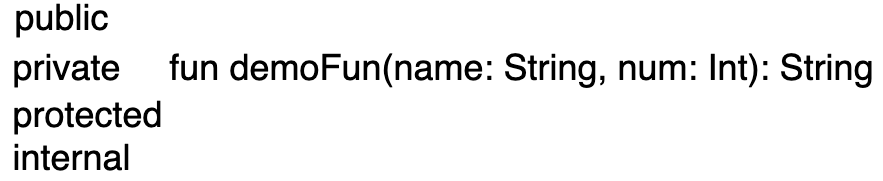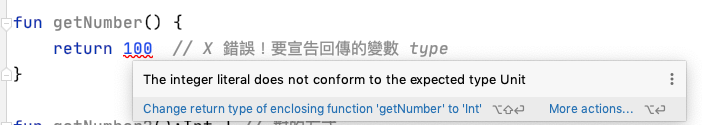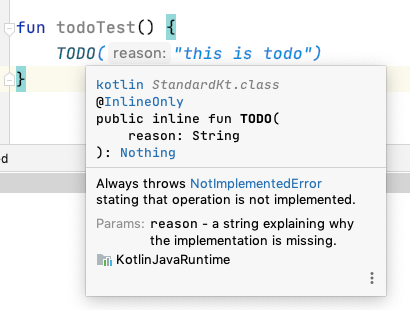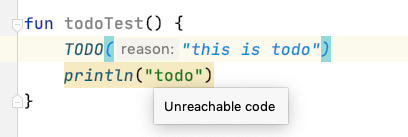## function 的結構• public: 其實可以不用寫，fun 預設就是 public，代表是隨處都可以看見
• private: 在同一 class 下可以看見
• protected: 在同一 class 或 class 的子 class 下可以看見
• internal: 在相同 module 下可以看見

fun - 代表 function 宣告

demoFun - function 名稱

(name: String，num: Int) - 這些是要傳入的參數和型態

:String - 最後是這個 function 要回傳的結果變數型態

## Ｍodule

• IntelliJ IDEA 模組;
• Maven 專案;
• Gradle 源集;
• 使用 Ant 任務呼叫編譯的一組檔案。

## 函數 (function)

``````fun getNumber2():Int { // 對的方式
return 100
}
``````### Unit 類型( function type)

Unit 就是我們在 Java 中常常看到的 void 的宣告，意思就是這個 function 不回傳值，為什麼要多一個 Unit 的型態來呢？ 因為在以往 Java 的時候，void 因為不是一個變數型態而無法做出相對應的泛型，所以 Kotlin 為了泛型的這個問題，才創造了這個變數型態，預設不寫，回傳值就是 Unit 類型

## single-expression function

``````fun getNumber3(): Int = 100

fun getNumber4() = 100

fun getNumber5(num: Int) = when (num) {
0 -> "it's zero"
in 1..99 -> "it's between 1 to 99"
else -> "not match"
}

println(getNumber5(1000)) // not match
``````

## function default value (預設值)

``````// default values
fun sum(a: Int, b: Int, c: Int = 10) = a + b + c

println(sum(1, 2, 3)) // 6
println(sum(1, 2))    // 13
``````

## named paramter (具名參數)

``````fun sum(a: Int, b: Int, c: Int = 10) = a + b + c

println(sum(b = 2, c = 1, a = 5)) // 8
``````

## function overloading (函數重載）

Kotlin 中 overloading 跟在 Java 一樣，function 名稱都一樣的狀況下，就是靠參數數量不同來區分

``````fun overload() {
println("overloading")
}
fun overload(num: Int) {
println("overloading num: \$num")
}
fun overload(num: Int, isTrue: Boolean) {
println("overloading num: \$num, is true: \$isTrue")
}

overload()  // overloading
overload(1)  // overloading num: 1
overload(2, false) // overloading num: 2，is true: false
overload(isTrue = true, num = 10) // overloading num: 10，is true: true
``````

## Nothing 類型

Nothing 類型其實類似 Unit，但不同的是，宣告 Nothing 類型，結果會拋出 Exception，為什麼要這樣做呢？ 其實我聽到會拋出 Exception...我是想，我應該這輩子不會使用吧XD## 反引號的 function name

``````@Test
fun `greet with Dolly returns 'Hello, Tim!'`() {
client.get().uri("/rest?name=Tim")
.exchange()
.expectStatus().isOk
.expectBody<Greeting>()
.consumeWith { assertEquals("Hello, Tim!", it.responseBody?.message) }
}
``````